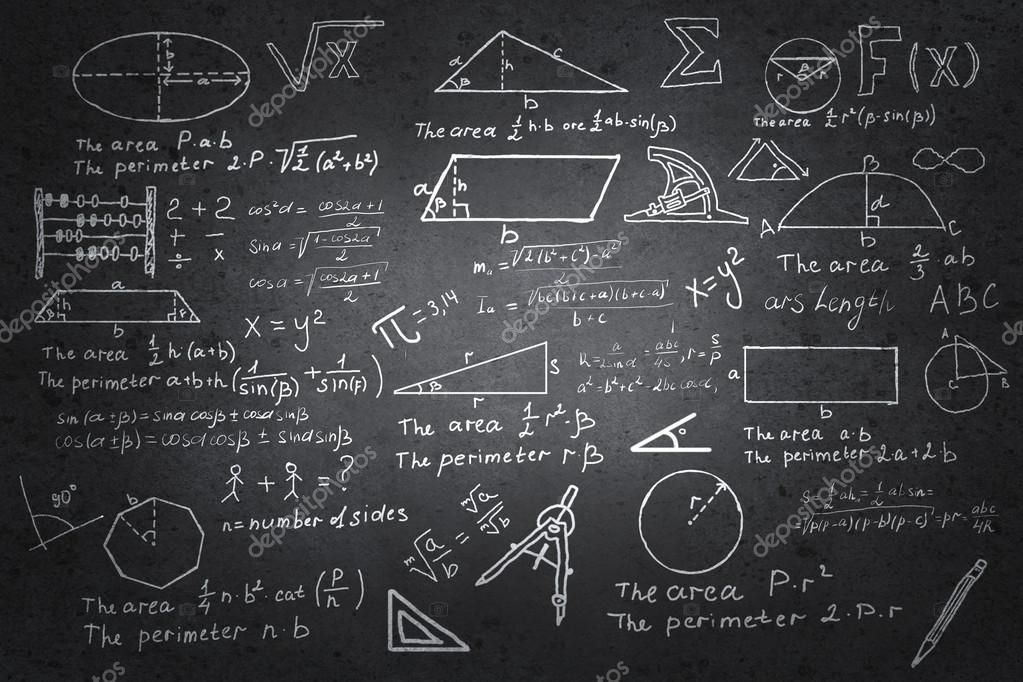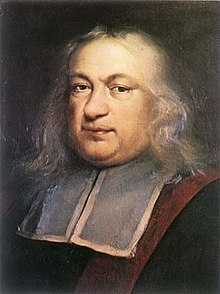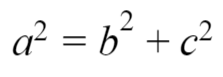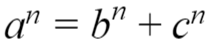es# Why did we solve Fermat’s Last Theorem and the value of irrelevant proofs

24.03.2020

In the year 1637, French mathematician Pierre de Fermat made a note in the margin of his copy of the Arithmetica:

"It is impossible to separate a cube into two cubes, or a fourth power into two fourth powers, or in general, any power higher than the second, into two like powers. I have discovered a truly marvelous proof of this, which this margin is too narrow to contain."

His Arithmetica was recovered once he died, and the note was found. So far so good. Just a small problem remained: no one was able to replicate Fermat's "marvelous proof". Time passed, and that short note continued to frustrate the world of Mathematics. It became known as Fermat's Last Theorem, and it would continue to do so for 357 years.On October 24, 1994, British mathematician Andrew Wiles published two papers that put the search to an end. Most of the greatest minds in Mathematics had given the problem a try. Everyone had failed until then. And yet, people had kept on trying until eventually one of them succeeded. In the end, the proof filled 129 pages, took three days for Wiles to explain and had required his undivided attention for seven years (not to mention it guided his entire career). But why did we do it? Why dedicate so much effort to such a short note? Why did we solve Fermat's Last Theorem?

Perhaps the Theorem was important, you might say. It could very well be that Fermat had presented us with one of the great questions in Mathematics, so great we couldn't afford to stop trying. After all, proving the Theorem led to a few big discoveries, particularly the modularity theorem. On closer inspection, however, we see that Fermat's Last Theorem did not lead to those proofs, but rather that those new theorems were necessary to prove Fermat's, which wasn't even important on its own either. But don't just take my word for it: Fermat's Last Theorem is so simple you can see that for yourself. Let us take a look at what it really says.

We have all heard of the Pythagorean Theorem. It's one of the first theorems we are ever taught in school, and the first one taught to us under that title. "In a right triangle, the square of the hypotenuse is equal to the sum of the squares of the other two sides". We usually see it under the equationwhere a is the length of the hypotenuse and b and c are the other two sides. We also know there are some integer solutions to this Theorem. For example, the numbers 3, 4 and 5 are a solution to this equation, since 25=16+9. Now, what Fermat proposed was just a small variation of this. He said that there are no integer solutions to the equationwhere n is an integer greater than two. So nothing too complicated, as you can see. He was just saying you can't find sets of number that behave like 3, 4 and 5 do for Pythagoras when you are working with bigger exponents.

So essentially mathematicians had spent more than three and a half centuries trying to solve an irrelevant variation of the Pythagorean Theorem. Just consider: when Fermat made his infamous note, king Louis XIV wasn't even born. When Wiles solved it, the Cold War had ended and the Soviet Union had fallen apart. So why do it at all? Was it a waste of time?

I would argue the "why" is very simple. I would argue that it doesn't matter if the Theorem was irrelevant or not because, in reality, theoretical mathematics are inherently irrelevant.

Mathematics stand out among the disciplines of Science for being nothing more than the purest pursuit of knowledge. It is so removed from the world one could even argue it's not a science at all. It's distinguishable from philosophy only in two things: the topics it studies and the unquestionability of its conclusions. It essentially consists of making rational deductions from a particular set of axioms, to the point that in Mathematics the real world is irrelevant. It does not matter whether a particular theorem is useful in practice or not, because mathematicians only care about whether it's true.

To borrow a particular example from G. H. Hardy , take Euclid's proof that there is an infinite number of primes. It is arguably the most important proof in mathematical history, as it serves as the cornerstone of arithmetics and number theory, a field so important that Gauss once said: "Mathematics is the queen of the sciences and number theory is the queen of Mathematics". The thing is, the amount of primes is irrelevant to anyone who is not a mathematician. An engineer will only ever use a finite amount of them, and for him it is enough to know that there are a lot, not necessarily an infinite amount. Even now, when the world of cryptography has somehow found use in number theory with the RSA code, "infinite" is more than they need. Like the engineer, they are happy with "a lot".

But of course Fermat's Last Theorem is different from Euclid's. After all, the latter is important in mathematics, while we have already seen that Fermat's isn't. But we have already mentioned the key goal of Mathematics: knowledge. The reason Euclid's Theorem is more important than Fermat's is because of the amount of proofs that need it to be true. It provides knowledge that allows us to reach more knowledge. But it still essentially answers to nothing more than the pursuit of knowledge, and so does Fermat's. Proving one or the other is still just a way to know more. So for me, proving Fermat's Last Theorem was worthwhile because it allowed us to know more than we did before.

And of course, there's another reason Fermat's Last Theorem got so much attention. "I have discovered a truly marvelous proof of this, which this margin is too narrow to contain." When Fermat wrote that, he was essentially writing a challenge. At first, people tried because they thought Fermat had done it. By the time we realised he probably hadn't managed to do it, it was too late. The problem had already become famous. It was suddenly an important conjecture, merely because of how many great minds had failed trying to solve it. It was even included in Hilbert's list of problems for the 20th century, in which Hilbert proposed the problems mathematicians should focus on for the next hundred years.

But to me this will always be a story about our irrational pursuit of rational knowledge. A story about truth and its inherent importance. A story about the beauty of mathematical discoveries. Why did we solve Fermat's Last Theorem? Because if we hadn't, we wouldn't know if it was true. And we are stubborn enough to count that as a good reason.

*REFERENCES

 H. Hardy, Godfrey. A Mathematician's Apology (Cambridge University Press: 1940). It is a book I recommend to anyone trying to understand what mathematics are really about.

JACOBO VILA RECIO